Question

# Question 4 of 18 (1 point) Attempt 2 of Unlimited View question. In a popuR! 7.1 Section Exercise 37-38 A sample...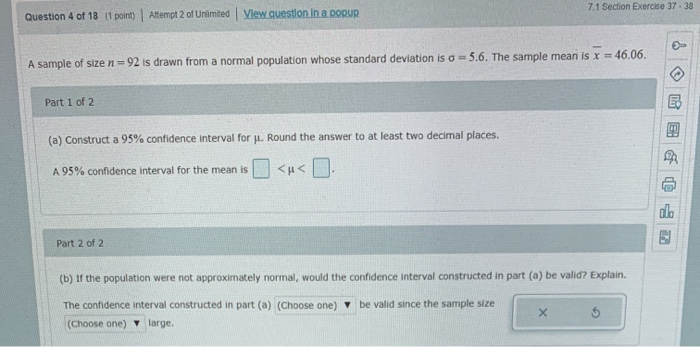Question 4 of 18 (1 point) Attempt 2 of Unlimited View question. In a popuR! 7.1 Section Exercise 37-38 A sample of size n = 92 is drawn from a normal population whose standard deviation is a = 5.6. The sample mean is x = 46.06. Part 1 of 2 (a) Construct a 95% confidence interval for Jl. Round the answer to at least two decimal places. A 95% confidence interval for the mean is <u< . Part 2 of 2 (b) If the population were not approximately normal, would the confidence interval constructed in part (a) be valid? Explain. The confidence interval constructed in part (a) (Choose one) y be valid since the sample size (Choose one) large.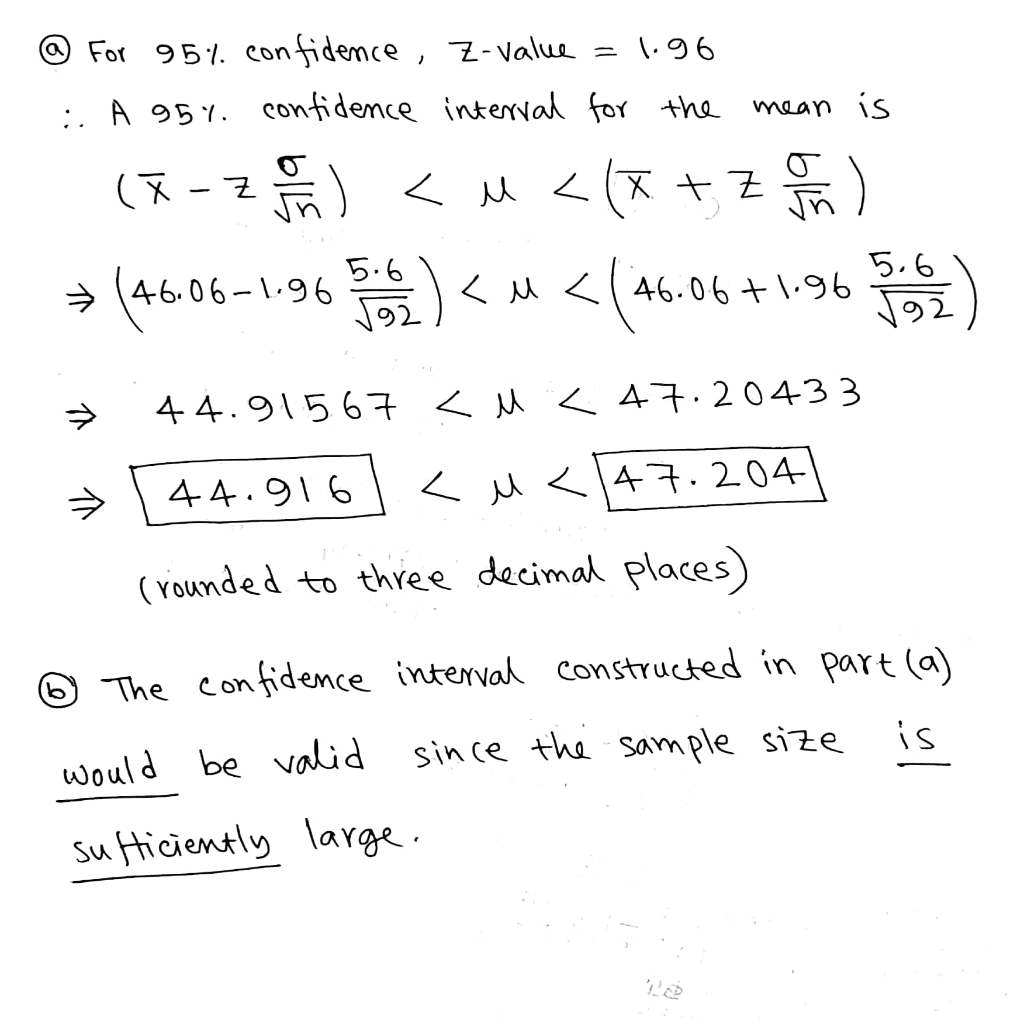#### Earn Coins

Coins can be redeemed for fabulous gifts.

Similar Homework Help Questions
• ### Question 1 of 13 (1 point) Attempt 1 of Unlimited View question in a popup 8.1...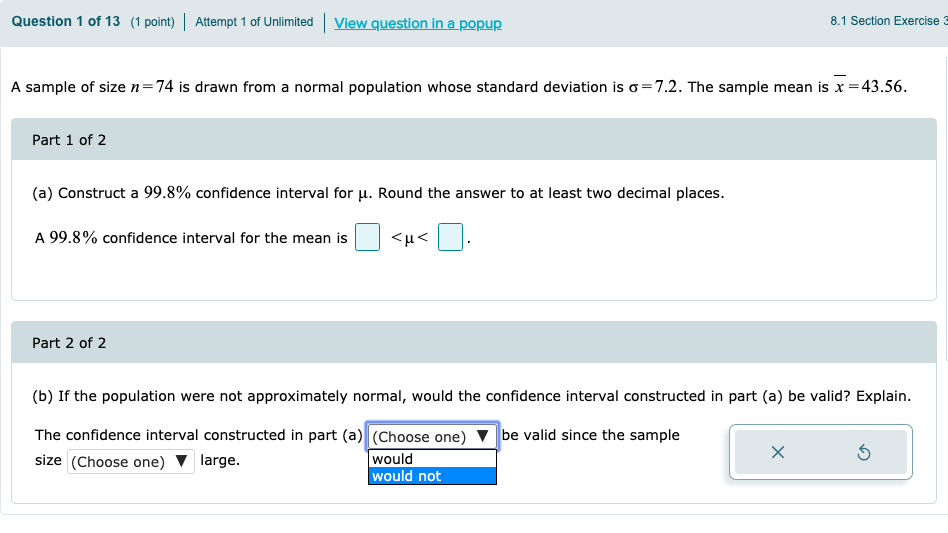Question 1 of 13 (1 point) Attempt 1 of Unlimited View question in a popup 8.1 Section Exercise A sample of size n=74 is drawn from a normal population whose standard deviation is o=7.2. The sample mean is x =43.56. Part 1 of 2 (a) Construct a 99.8% confidence interval for u. Round the answer to at least two decimal places. A 99.8% confidence interval for the mean is <u< . Part 2 of 2 (b) If the population were...

• ### 7.1 Section Exercise Question 3 of 18 (1 point) Altempt 3 of Unlimited View questlon in...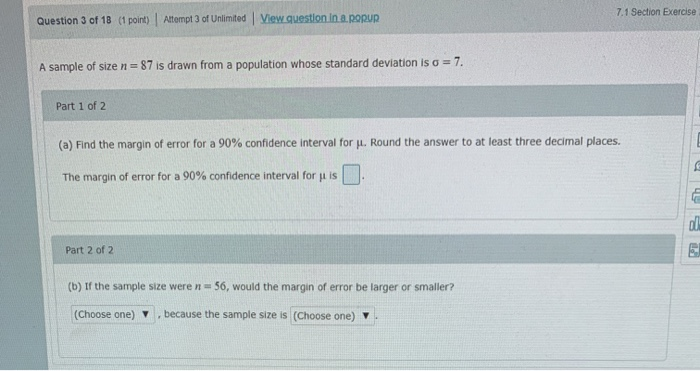7.1 Section Exercise Question 3 of 18 (1 point) Altempt 3 of Unlimited View questlon in a popup 7 A sample of size n 87 is drawn from a population whose standard deviation is a Part 1 of 2 (a) Find the margin of error for a 90% confidence interval for . Round the answer to at least three decimal places. The margin of error for a 90% confidence interval for u is Part 2 of 2 (b) If the...

• ### Please solve: Question 3 of 16 (15 points) I Attempt 1 of 1 View question in...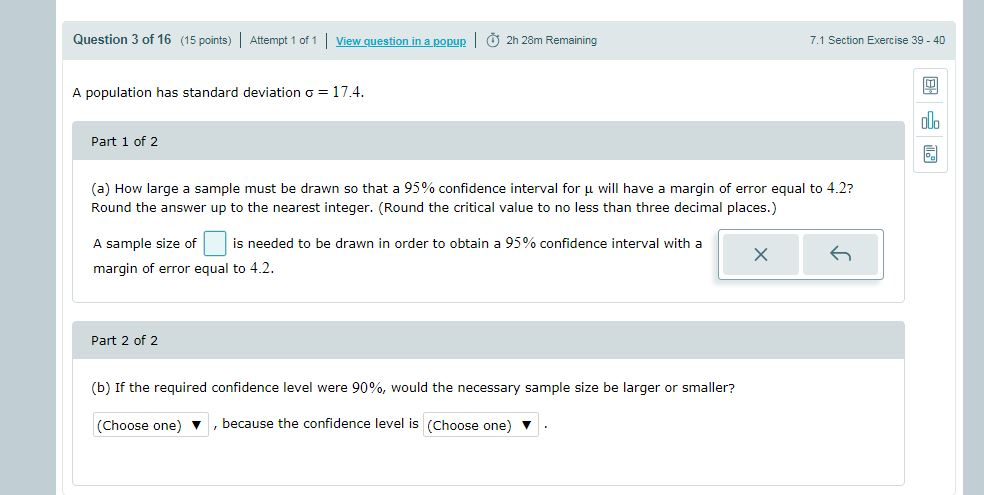Please solve: Question 3 of 16 (15 points) I Attempt 1 of 1 View question in a popup | 卤 2h 28m Remaining 7.1 Section Exercise 39-40 A population has standard deviation 17.4. alo Part 1 of 2 (a) How large a sample must be drawn so that a 95% confidence interval for μ will have a margin of error equal to 4.2? Round the answer up to the nearest integer. (Round the critical value to no less than three...

• ### Question 1 of 7 (1 point) Attempt 1 of 1 View question in a pour och...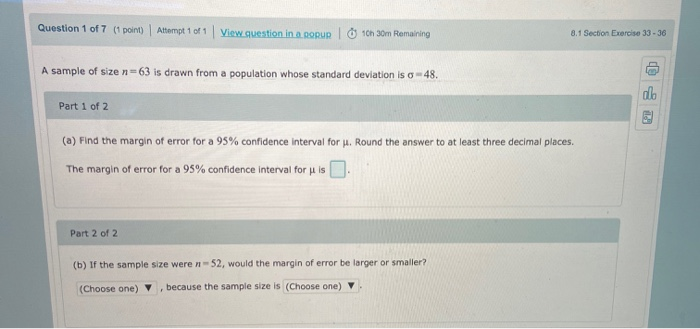Question 1 of 7 (1 point) Attempt 1 of 1 View question in a pour och 30m Remaining 8.1 Section Exercise 33-36 A sample of size n=63 is drawn from a population whose standard deviation is o - 48. co Part 1 of 2 (a) Find the margin of error for a 95% confidence interval for J. Round the answer to at least three decimal places. The margin of error for a 95% confidence interval for u is Part 2...

• ### Question 7 of 9 (1 point) View problem in a pop-up 7.1 Section Exercise 11 For...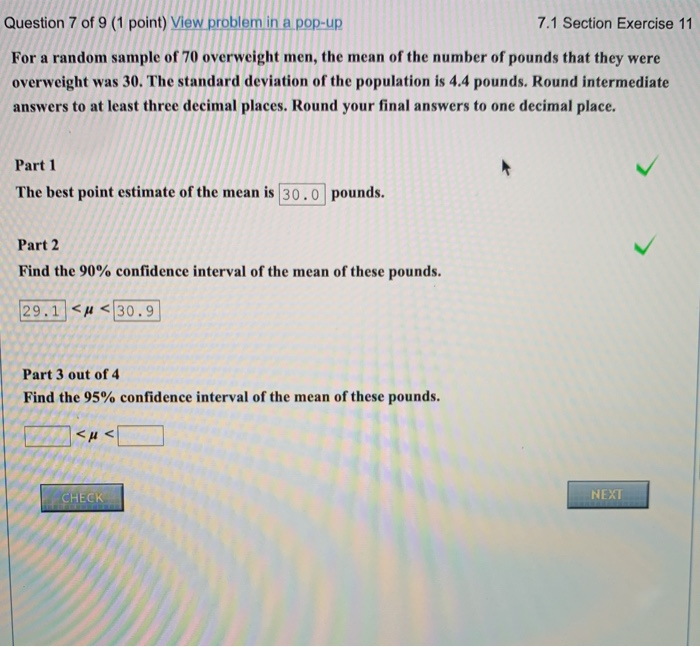Question 7 of 9 (1 point) View problem in a pop-up 7.1 Section Exercise 11 For a random sample of 70 overweight men, the mean of the number of pounds that they were overweight was 30. The standard deviation of the population is 4.4 pounds. Round intermediate answers to at least three decimal places. Round your final answers to one decimal place. Part 1 The best point estimate of the mean is 30.0 pounds. Part 2 Find the 90% confidence...

• ### Question 16 of 18 (1 point) Attempt 1 of Unlimited 74 Section Exercise 12 (cal Ages...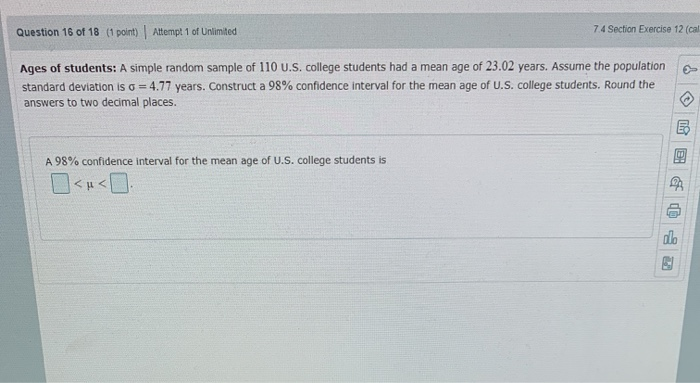Question 16 of 18 (1 point) Attempt 1 of Unlimited 74 Section Exercise 12 (cal Ages of students: A simple random sample of 110 U.S. college students had a mean age of 23.02 years. Assume the population standard deviation is o = 4.77 years. Construct a 98% confidence interval for the mean age of U.S. college students. Round the answers to two decimal places. A 98% confidence interval for the mean age of U.S. college students is

• ### Question 8 of 18 (1 point) Attempt 1 of Unlimited View question in a popur! 72...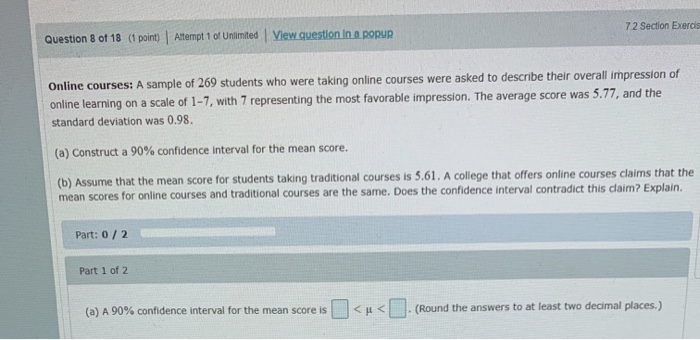Question 8 of 18 (1 point) Attempt 1 of Unlimited View question in a popur! 72 Section Exerci Online courses: A sample of 269 students who were taking online courses were asked to describe their overall impression of online learning on a scale of 1-7, with 7 representing the most favorable impression. The average score was 5.77, and the standard deviation was 0.98. (a) Construct a 90% confidence interval for the mean score. (b) Assume that the mean score for...

• ### Question 7 of 9 (1 point) View problem in a pop-up 7.1 Section Exercise 11 For...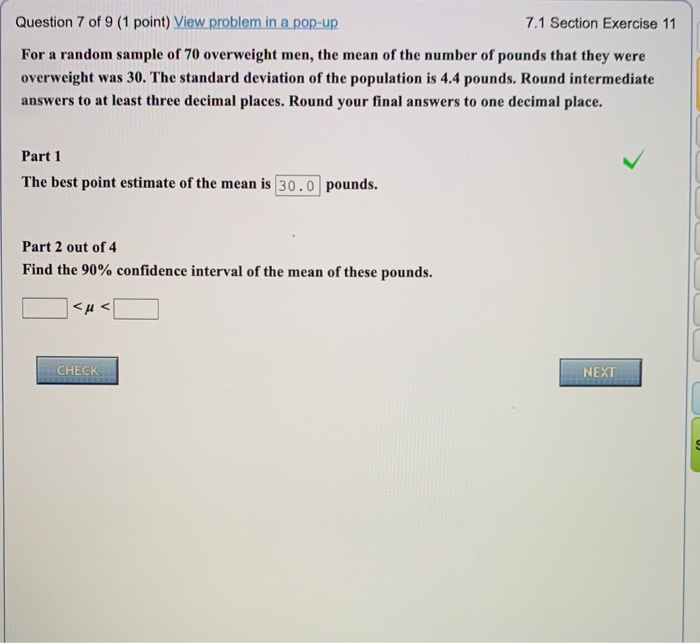Question 7 of 9 (1 point) View problem in a pop-up 7.1 Section Exercise 11 For a random sample of 70 overweight men, the mean of the number of pounds that they were overweight was 30. The standard deviation of the population is 4.4 pounds. Round intermediate answers to at least three decimal places. Round your final answers to one decimal place. Part 1 The best point estimate of the mean is 30.0 pounds. Part 2 out of 4 Find...

• ### Part 2 of 2 Lager or smaller.... because the sample size is smaller or larger Question...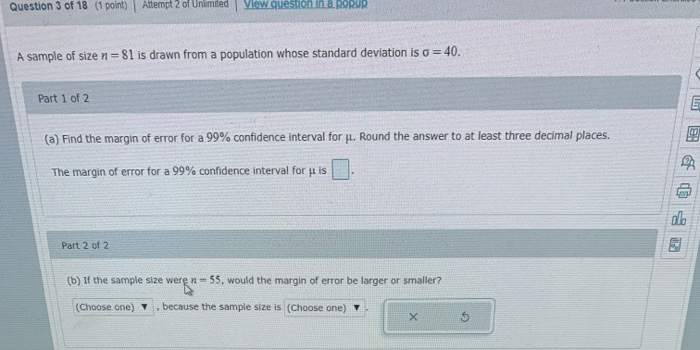Part 2 of 2 Lager or smaller.... because the sample size is smaller or larger Question 3 of 18 (1 point) Attempt 2 of Unlimited View question in a RORUR A sample of size n=81 is drawn from a population whose standard deviation is o = 40. Part 1 of 2 (a) Find the margin of error for a 99% confidence interval for u. Round the answer to at least three decimal places. The margin of error for a 99%...

• ### Question 7 of 1- 1 point) Attempt 1 of Unlimited View question in a popup 8.2...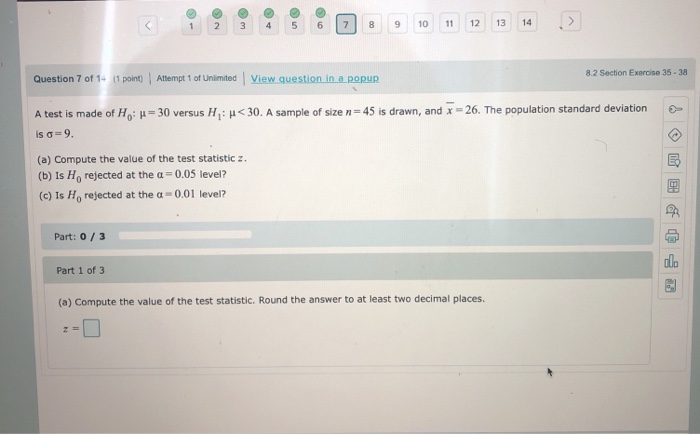Question 7 of 1- 1 point) Attempt 1 of Unlimited View question in a popup 8.2 Section Exercise 35-38 = 30 versus H: <30. A sample of size n=45 is drawn, and x = 26. The population standard deviation A test is made of H, is 9. (a) Compute the value of the test statistic z. (b) is Ho rejected at the a0.05 level? (c) Is He rejected at the c = 0.01 level? Part: 0/3 Part 1 of 3...